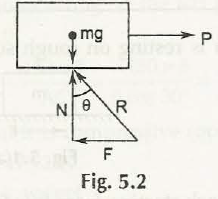This angle of friction is best defined with this figure:In the figure above, you can find an angle made with normal reaction or N and the force by friction or F. As a result you will get an equation:

Tan ϕ = F /N which is then equal to µ N / N = µ

So we get the angle of friction, ϕ = tan -1 µ

Links of Next Mechanical Engineering Topics:-### Customer Reviews

My Homework Help
Rated 5.0 out of 5 based on 510 customer reviews at